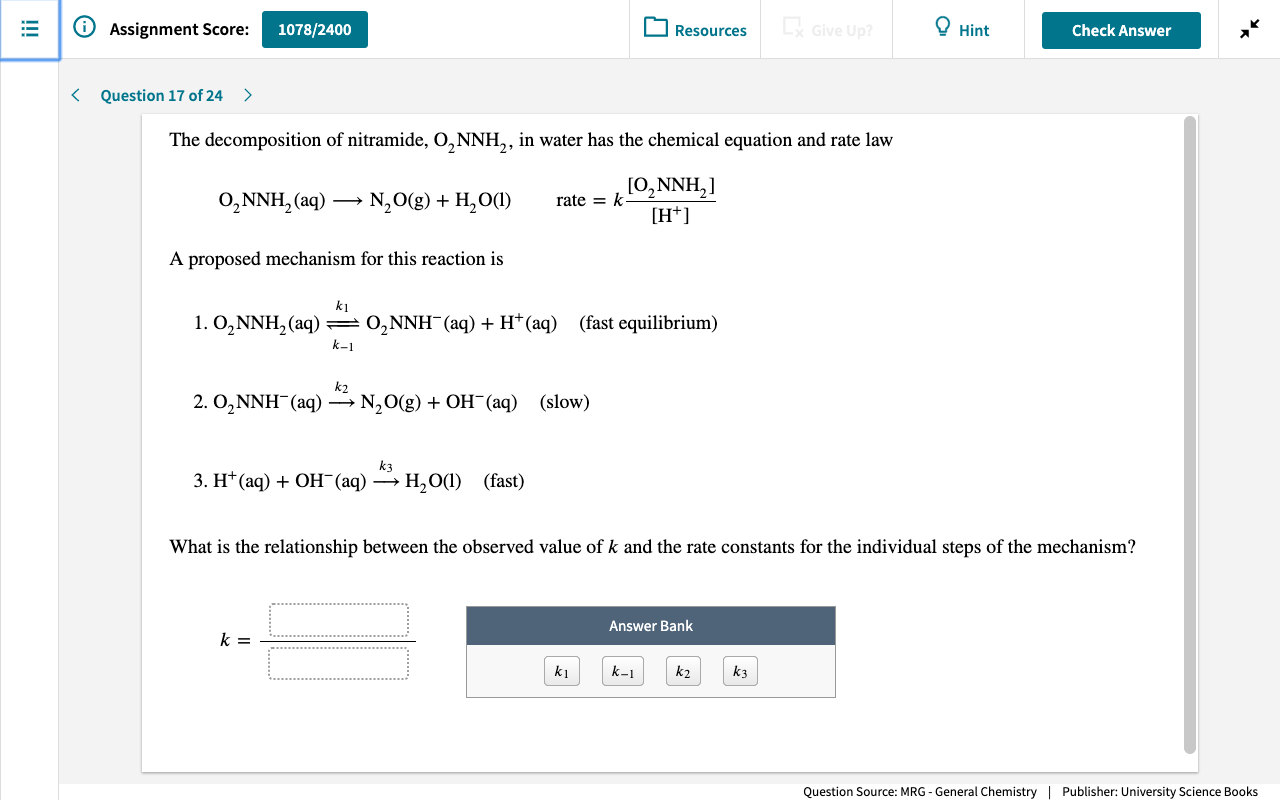# The decomposition of nitramide, O2NNH2, in water has the chemical equation and rate law O2NNH2(aq) → N2O(g) + H2O(l) rate = k[O2NNH2]/[H+] A proposed mechanism for this reaction is 1. O2NNH2 ⇌ O2NNH-(aq) + H+(aq) (fast equilibrium) 2. O2NNH-(aq) → N2O(g) + OH-(aq) (slow) 3. H+(aq) + OH-(aq) → H2O(l) (fast) What is the relationship between the observed value of k and the rate constants for the individual steps of the mechanism?